# Moonshine conjectures

(diff) ← Older revision | Latest revision (diff) | Newer revision → (diff)

In 1978, J. McKay observed that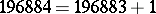. The number on the left is the first non-trivial coefficient of the-function, and the numbers on the right are the dimensions of the smallest irreducible representations of the Fischer–Griess Monster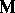[a10] (cf. also Sporadic simple group). On the one side stands a modular function; on the other, a finite sporadic simple group. Moonshine is the explanation and generalization of this unlikely connection.

Monstrous moonshine [a6] conjectured that there is an infinite-dimensional graded vector space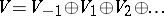, with the following properties. Each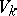carries a finite-dimensional representation of; write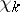for its character. For each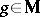, define the Thompson–McKay series, where. Thenis a generator ( "Hauptmodul" ) of the field of modular functions for some genus-group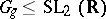. The group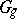contains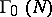as a normal subgroup, wheredivides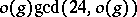(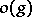is the order of).

There aredistinct Thompson series (has onlyconjugacy classes). For example,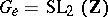and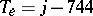, where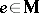is the identity.has two order-conjugacy classes, corresponding to the modular groups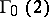andLet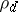denote the-dimensional irreducible representation of; then,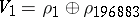, and.

Central to these conjectures is the moonshine module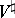, constructed in [a9]. It is an important example of a vertex operator algebra (VOA) [a2], [a9], and as such possesses infinitely many heavily constrained bilinear products. One of these products makes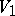into the Griess algebra [a10] extended by an identity element. The automorphism group ofis.

The monstrous moonshine conjectures, and, in particular, the identification ofwith, were proved in [a4], which also defined vertex operator algebras and a generalization of Kac–Moody algebras called Borcherds or generalized Kac–Moody algebras [a3] (cf. also Kac–Moody algebra; Borcherds Lie algebra).

The series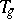were generalized by S.P. Norton to commuting pairs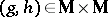[a12]. In particular, to each such pair there is a modular function, invariant under a genus-group, such that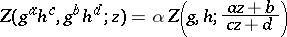for some root of unity, for any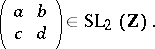This action of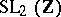is related to its natural action on the fundamental groupof the torus. The coefficients of the-expansion of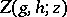are characters of the centralizerevaluated at. Simultaneous conjugation of,leaves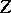unchanged: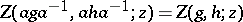. The Thompson–McKay series are recovered by the specialization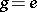: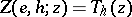. Only special cases of these generalized moonshine conjectures have been proven.

There are several other conjectures. For example, the series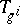were conjecturally related by the replication formulas [a6], [a1]: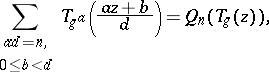(a1)

where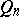is the unique polynomial for which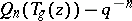is a power series with only strictly positive powers of. The resemblance of (a1) with Hecke operators is not accidental. These formulas are related to identities such as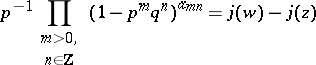(a2)

for, where, as well as to the existence of several modular equations obeyed by the. These identities played a large role in the proof of moonshine. R. Borcherds proved that theobey (a1) by using the denominator identities of Borcherds algebras; the resulting modular equations can be used to prove the Hauptmodul property [a7]. It would be very nice to see other direct connections between the group operation inand the series: for instance, are there other relations among thewhich correspond to more general products of conjugacy classes in?

The Hirzebruch prize question [a11] asks for a compact, differentiable,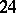-dimensional manifold on whichacts by diffeomorphisms and whose twisted Witten genus is. It is still open (as of 2000).

Vertex operator algebras are a key component in moonshine. There will be some form of moonshine for finite subgroups of the automorphism group of any rational vertex operator algebra obeying a technical (and probably redundant) "C2 condition" (see e.g. [a18]). The resulting functions, however, will not be Hauptmoduls in general. There is no complete understanding yet (2000) of why Hauptmoduls are associated to, although it has been conjectured to do with the "6-transposition property" of[a12], or the uniqueness of[a17].

Moonshine is related to conformal field theory (e.g.is the chiral algebra of a holomorphictheory), in which context many of moonshine's special features appear natural [a8], [a17], and this has motivated some of the developments (most significantly the definition of vertex operator algebras).

It still (as of 2000) is unclear why certain Hauptmoduls appear in moonshine and others do not. What form does moonshine (e.g. the replication formulas) take in genus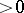(see e.g. [a16])? How about moonshine for other groups (see e.g. [a13])? In [a14], a variant called modular moonshine was established, whereis replaced with vertex algebras over characteristic, and where the role ofis taken by centralizers inof orderelements.

For his work in monstrous moonshine and related topics, Borcherds was awarded a Fields Medal in 1998 [a5].

How to Cite This Entry:
Moonshine conjectures. Encyclopedia of Mathematics. URL: http://encyclopediaofmath.org/index.php?title=Moonshine_conjectures&oldid=18566
This article was adapted from an original article by Terry Gannon (originator), which appeared in Encyclopedia of Mathematics - ISBN 1402006098. See original article